# Trigonometry

#### Prev     Next

Trigonometry:

 Trigonometry: In a right angled OAB, where ∠BOA = θ ,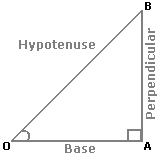i. sin(θ) = Perpendicular / Hypotenuse     = AB / OB ;

ii. cos(θ) = Base / Hypotenuse  = OA / OB ;

iii. tan(θ) = Perpendicular / Base = AB / OA ;

iv. cosec(θ)= 1 / sin(θ) = OB / AB ;

v. sec(θ) = 1 / cos(θ) = OB / OA ;

vi. cot(θ) = 1 / tan(θ) = OA / AB ;

 Trigonometrical Identities:

i. sin2 (θ) + cos2 (θ) = 1.
ii. 1 + tan2 (θ) = sec2 (θ) .
iii. 1 + cot2 (θ) = cosec2 (θ) .

Values of T-ratios: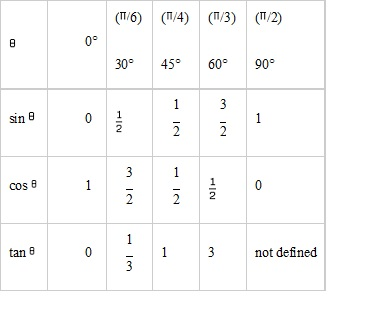 Angle of Elevation: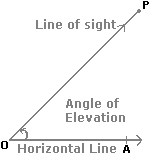Suppose a man from a point O looks up at an object P, placed above the level of his eye. Then, the angle which the line of sight makes with the horizontal through O, is called the angle of elevation of P as seen from O.
Angle of elevation of P from O = ∠AOP.

 Angle of Depression: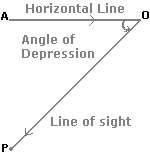Suppose a man from a point O looks down at an object P, placed below the level of his eye, then the angle which the line of sight makes with the horizontal through O, is called the angle of depression of P as seen from O.

# Aptitude Questions (Trigonometry):

1. The maximum and minimum value of 8 sin θ and cos θ + 4 cos 2 θ is:

A)    4 and -4

B)    16 and -16

C)    4√2 and -4√2

D)    8 and -8

E)    None of these

Explanation:
Here, 8 sin(θ) cos(θ) + 4 cos 2(θ) = 4 sin 2(θ) + 4 cos 2(θ)
So, maximum value =√(42+42) = 4√2 and minimum value = -√(42+42) = -4√2

2. Solve cos θ + sin θ = √2, then the value of θ is:

A)    π/3

B)    π/4

C)    π/6

D)    π/2

E)    None of these

Explanation:
cos(θ)+ sin(θ) = √2
cos(θ)/ √2 + sin(θ)/ √2 = 1 (∵ divided throughout by √2)
=> cos(θ) cos(45º) + sin(θ) sin(45º)= 1
cos( θ-45º) = 1 = cos0
∴ (θ – 45º ) = 0
=> θ =  45º = π/4

3. The value of  (sin135º– cos120º) / (sin135º+cos120º)  is

A)    2 + 3√2

B)    2 – 3√2

C)    3 – 2√2

D)    3 + 2√2

E)    None of these

Explanation: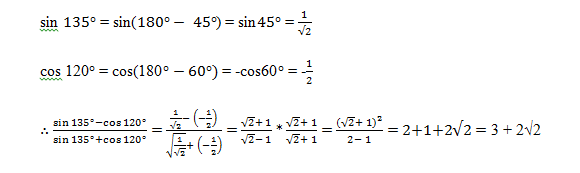4. The value of is (tan47º + tan43º) / (1-tan47ºtan43º) is

A)    1

B)    ∝

C)    0

D)    -1

E)    None of these

Explanation:
As, (tan A + tan B) / (1-tan A tan B) = tan(A + B)
=(tan47º + tan43º) / (1-tan47ºtan43º) = tan(47° + 43°) = tan90° =

5. If A – B = π/3, then the value of cos A cos B + sin A sin B is

A)    ½

B)    1

C)    3/2

D)    0

E)    None of these